﻿ 大规模MIMO系统下的全向STBC编码设计与性能分析

# 大规模MIMO系统下的全向STBC编码设计与性能分析Design and Performance Analysis of Omnidirectional Space-Time Block Code in Massive MIMO Systems

Abstract: Common signals should be transmitted omnidirectionally to guarantee cell-wide coverage in massive MIMO systems under celluar communication units. Space-Time Block Code (STBC) has received widespread application in MIMO systems, in this paper, we focus on the omnidirectional STBC in massive MIMO downlink, which provides a new thinking for reducing the burden of downlink pilot overhead. We firstly design an omnidirectional precoding (OP) matrix for 2*2 Alamouti Code, which guarantees equal receiving power for user terminals in all spatial directions at any instant time as well as equal instantaneous power for each transmitting antenna. To obtain diversity order of four, OP matrices utilizing $4*4$ STBC and OSTBC Code are also proposed, respectively. What’s more, the condition of OP matrices is rewritten considering constant angle domain, and several examples of OP matrices are proposed. We analyse the system performance utilizing omnidirectional STBC in four aspects: bit error rate, peak-to-average ratio (PAPR), channel capacity and outage probability. Simulation results show that one proposed design can 1) reduce bit error bit; 2) maximize channel capacity; 3) preserve the PAPR of transmitted signals.

1. 引言

2. STBC编码下的MIMO系统模型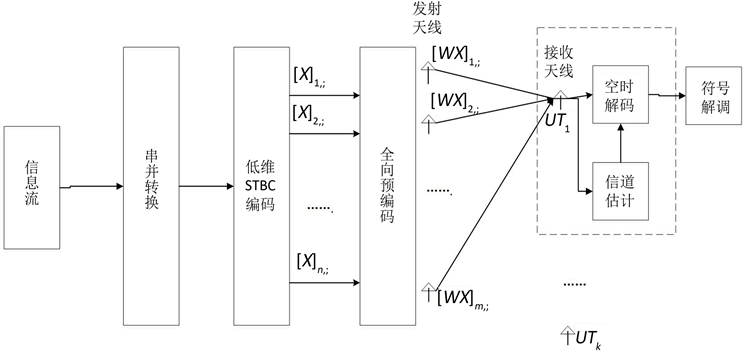Figure 1. System model of STBC coded massive MIMO systems

$\left[{y}_{k,1},{y}_{k,2},\cdots ,{y}_{k,T}\right]={h}_{k}^{\text{T}}S+\left[{z}_{k,1},{z}_{k,2},\cdots ,{z}_{k,T}\right]$ (1)

${P}_{k}=\mathbb{E}\left\{{|{h}_{k}W{x}_{t}|}^{2}\right\}={x}_{t}^{H}{W}^{H}{F}_{M}^{H}{\Lambda }_{k}{F}_{M}W{x}_{t}$ (2)

·全向传输条件：首先，所设计的OP矩阵需要满足在$M$个离散角度域上具有相同的传输功率，即列向量 ${F}_{M}W{x}_{t}$ 的M个分量具有相同的幅值： ${\left[{F}_{M}W{x}_{t}\right]}_{1}={\left[{F}_{M}W{x}_{t}\right]}_{2}=\cdots ={\left[{F}_{M}W{x}_{t}\right]}_{M}=c$ ，此时容易验证K个接收天线上所接收的信号功率 $P\left({\Lambda }_{1}\right)=P\left({\Lambda }_{2}\right)=\cdots =P\left({\Lambda }_{K}\right)=M{c}^{2}$ 为定值，符合设计要求。

·PA利用率条件：为达到较高的天线功放利用率，我们考虑保证M个发送天线上的传输信号相等，即对任一时刻t，均有 ${\left[W{x}_{t}\right]}_{1}={\left[W{x}_{t}\right]}_{2}=\cdots ={\left[W{x}_{t}\right]}_{M}$ ，由各发送天线上的瞬时功率与OP矩阵 $W$ 和STBC矩阵 $X$ 都有关，因此本文将STBC矩阵中的传输符号与预编码矩阵联合设计。

3. 离散角度下的全向STBC编码设计

3.1. N = 2时的全向STBC编码设计

$N=2$ 时，我们使用Alamouti码作为低维码，则有 $N=T=2$ 。Alamouti码是一种发射天线数为2，可以提供满分集速率的正交空时码，编码器输出的两个符号在两个时间周期内被发送出去，如下式所示：

${X}_{A}=\left[\begin{array}{cc}{x}_{1}& {x}_{2}^{*}\\ {x}_{2}& -{x}_{1}^{*}\end{array}\right]$ (3)

$W=\text{diag}\left({\Pi }_{r}{F}_{M}c\right)\left({1}_{M/2}\otimes P\right)$ (4)

${\left[c\right]}_{m}=\left\{\begin{array}{l}{\text{e}}^{j\text{π}\gamma {m}^{2}/M}\text{}\text{\hspace{0.17em}}\text{\hspace{0.17em}}\text{\hspace{0.17em}}\text{\hspace{0.17em}}M\text{iseven}\\ {\text{e}}^{j\text{π}\gamma m\left(m+1\right)/M}\text{}M\text{isodd}\end{array}$ (5)

${\Pi }_{k}=\left[\begin{array}{cc}0& {I}_{k}\\ {I}_{M-k}& 0\end{array}\right]$ (6)

${p}_{11}{p}_{12}^{\ast }\in ℝ$${p}_{21}{p}_{22}^{\ast }\in ℝ$

${|{p}_{11}|}^{2}+{|{p}_{12}|}^{2}={|{p}_{21}|}^{2}+{|{p}_{22}|}^{2}$

③ 矩阵 $P$ 是规范列正交的。

$\left[c{\Pi }_{r}\left(1\right),c{\Pi }_{r}\left(2\right),\cdots ,c{\Pi }_{r}\left(2{M}_{0}-1\right)\right]\stackrel{DFT}{\to }\left[d\left(0\right),d\left(1\right),\cdots ,d\left(2{M}_{0}-1\right)\right]$ (7)

$d\left(k\right)=\underset{n=0}{\overset{2{M}_{0}-1}{\sum }}c{\Pi }_{r}\left(n\right){\text{e}}^{-2\text{π}jkn/2{M}_{0}}$ (8)

$={\text{e}}^{-j\text{π}{\left(n-r\right)}^{2}/2{M}_{0}}\underset{n=0}{\overset{2{M}_{0}-1}{\sum }}{\text{e}}^{2j\text{π}{\left(n-k-r\right)}^{2}/2{M}_{0}}$ (9)

$=\underset{n=0}{\overset{2{M}_{0}-1}{\sum }}{\text{e}}^{j\text{π}{\left(n-r\right)}^{2}/2{M}_{0}}{\text{e}}^{-2\text{π}jkn/2{M}_{0}}$ (10)

${S}_{A}=\left[\begin{array}{cc}d\left(0\right){p}_{11}& d\left(0\right){p}_{12}\\ d\left(1\right){p}_{21}& d\left(1\right){p}_{22}\\ d\left(2\right){p}_{11}& d\left(2\right){p}_{12}\\ ⋮& ⋮\\ d\left(2{M}_{0}-1\right){p}_{21}& d\left(2{M}_{0}-1\right){p}_{22}\end{array}\right]\left[\begin{array}{cc}{x}_{1}& {x}_{2}^{*}\\ {x}_{2}& -{x}_{1}^{*}\end{array}\right]$ (11)

${F}_{M}W{x}_{t}={F}_{M}\left[\begin{array}{c}\left({p}_{11}{x}_{1}+{p}_{12}{x}_{2}\right)d\left(0\right)\\ \left({p}_{21}{x}_{1}+{p}_{22}{x}_{2}\right)d\left(1\right)\\ \left({p}_{11}{x}_{1}+{p}_{12}{x}_{2}\right)d\left(2\right)\\ ⋮\\ \left({p}_{21}{x}_{1}+{p}_{22}{x}_{2}\right)d\left(2{M}_{0}-1\right)\end{array}\right]$ (12)

$\begin{array}{c}{F}_{M}W{x}_{1}\left(k\right)=\left({p}_{11}{x}_{1}+{p}_{12}{x}_{2}\right)\underset{n=0}{\overset{2{M}_{0}-1}{\sum }}\frac{d\left(n\right)+{\left(-1\right)}^{n}d\left(n\right)}{2}{W}_{2{M}_{0}}^{kn}\\ \text{\hspace{0.17em}}\text{\hspace{0.17em}}+\left({p}_{21}{x}_{1}+{p}_{22}{x}_{2}\right)\underset{n=0}{\overset{2{M}_{0}-1}{\sum }}\frac{d\left(n\right)-{\left(-1\right)}^{n}d\left(n\right)}{2}{W}_{2{M}_{0}}^{kn}\text{ }\text{ }\text{ }\text{ }\text{ }\text{ }\text{ }\text{ }\text{ }\text{ }\text{ }\text{ }\text{(13)}\text{ }\\ =\left({p}_{11}{x}_{1}+{p}_{12}{x}_{2}\right)\frac{X\left(k\right)+X\left({\left(k+{M}_{0}\right)}_{2{M}_{0}}\right)}{2}\\ \text{\hspace{0.17em}}\text{\hspace{0.17em}}+\left({p}_{21}{x}_{1}+{p}_{22}{x}_{2}\right)\frac{X\left(k\right)-X\left({\left(k+{M}_{0}\right)}_{2{M}_{0}}\right)}{2}\text{ }\text{ }\text{ }\text{ }\text{ }\text{ }\text{ }\text{ }\text{ }\text{ }\text{ }\text{ }\text{(14)}\\ ={f}_{1}\left({x}_{1},{x}_{2}\right)X\left(k\right)+{f}_{2}\left({x}_{1},{x}_{2}\right)X\left({\left(k+{M}_{0}\right)}_{2{M}_{0}}\right)\text{ }\text{ }\text{ }\text{ }\text{ }\text{ }\text{ }\text{ }\text{ }\text{ }\text{ }\text{ }\text{(15)}\end{array}$

$\begin{array}{l}{|{F}_{M}W{x}_{1}\left(k\right)|}^{2}=\left({|{f}_{1}\left({x}_{1},{x}_{2}\right)|}^{2}+{|{f}_{2}\left({x}_{1},{x}_{2}\right)|}^{2}\right){\lambda }^{2}\left(2{M}_{0}\right)\\ \text{}\text{\hspace{0.17em}}\text{\hspace{0.17em}}\text{\hspace{0.17em}}+2\Re \left({f}_{1}\left({x}_{1},{x}_{2}\right){f}_{2}^{*}\left({x}_{1},{x}_{2}\right)X\left(k\right){X}^{*}\left({\left(k+{M}_{0}\right)}_{2{M}_{0}}\right)\right)\end{array}$ (16)

$\frac{{|W{x}_{1}\left(k\right)|}^{2}}{{\lambda }^{2}\left(2{M}_{0}\right)}={|{p}_{11}|}^{2}{|{x}_{1}|}^{2}+{|{p}_{12}|}^{2}{|{x}_{2}|}^{2}+2\Re \left({p}_{11}{p}_{12}^{*}{x}_{1}{x}_{2}^{*}\right)\text{,}k\equiv 0\left(\mathrm{mod}2\right)$ (17)

$\frac{{|W{x}_{1}\left(k\right)|}^{2}}{{\lambda }^{2}\left(2{M}_{0}\right)}={|{p}_{21}|}^{2}{|{x}_{1}|}^{2}+{|{p}_{22}|}^{2}{|{x}_{2}|}^{2}+2\Re \left({p}_{21}{p}_{22}^{*}{x}_{1}{x}_{2}^{*}\right),\text{}k\equiv 1\left(\mathrm{mod}2\right)$ (18)

$\left\{\begin{array}{l}{x}_{1}={\text{e}}^{j\mathrm{sin}2\text{π}n/T}\text{}\\ {x}_{2}=j{\text{e}}^{-j\mathrm{sin}2\text{π}n/T}\end{array}$ (19)

3.2. N = 4时采用星座旋转编码的全向STBC编码设计

${X}_{J}=\left[\begin{array}{cccc}{x}_{1}& -{x}_{2}^{*}& -{\left({x}_{3}{\text{e}}^{j\theta }\right)}^{*}& {x}_{4}{\text{e}}^{j\theta }\\ {x}_{2}& {x}_{1}^{*}& -{\left({x}_{4}{\text{e}}^{j\theta }\right)}^{*}& -{x}_{3}{\text{e}}^{j\theta }\\ {x}_{3}{\text{e}}^{j\theta }& -{\left({x}_{4}{\text{e}}^{j\theta }\right)}^{*}& {x}_{1}^{*}& -{x}_{2}\\ {x}_{4}{\text{e}}^{j\theta }& {\left({x}_{3}{\text{e}}^{j\theta }\right)}^{*}& {x}_{2}^{*}& {x}_{1}\end{array}\right]$ (20)

${W}_{J}=\text{diag}\left({\Pi }_{r}{F}_{M}c\right)\left({1}_{M/4}\otimes \left({I}_{2}\otimes {H}_{2}\right)\right)$ (21)

$\left\{\begin{array}{l}{\varphi }_{1}\left(n\right)=\frac{1}{D}\underset{i=0}{\overset{D-1}{\sum }}{\epsilon }_{i}^{n}\text{}\\ {\varphi }_{2}\left(n\right)=\frac{1}{D}\underset{i=0}{\overset{D-1}{\sum }}{\epsilon }_{i}^{n-\left(D-1\right)}\\ \text{\hspace{0.17em}}\text{\hspace{0.17em}}\text{\hspace{0.17em}}\text{\hspace{0.17em}}\text{\hspace{0.17em}}\text{\hspace{0.17em}}\text{\hspace{0.17em}}\text{\hspace{0.17em}}\text{ }\text{ }\text{\hspace{0.17em}}⋮\\ {\varphi }_{D}\left(n\right)=\frac{1}{D}\underset{i=0}{\overset{D-1}{\sum }}{\epsilon }_{i}^{n-1}\end{array}$ (22)

${F}_{M}{W}_{J}{x}_{1}\left(k\right)=\underset{j=1}{\overset{4}{\sum }}{\left[Q\right]}_{j,;}{x}_{1}\underset{n=0}{\overset{4{M}_{0}-1}{\sum }}d\left(n\right){\varphi }_{j}\left(n\right){W}_{4{M}_{0}}^{kn}$ (23)

$\underset{n=0}{\overset{4{M}_{0}-1}{\sum }}d\left(n\right){\varphi }_{j}\left(n\right){W}_{4{M}_{0}}^{kn}=\frac{\underset{p=1}{\overset{4}{\sum }}{\epsilon }_{p-1}^{-j}X\left({\left(k+\left(j-1\right){M}_{0}\right)}_{4{M}_{0}}\right)}{D}$ (24)

$\begin{array}{c}{|{F}_{M}{W}_{J}{x}_{1}\left(k\right)|}^{2}={|\underset{i=1}{\overset{4}{\sum }}{f}_{i}\left({x}_{1},{x}_{2},{x}_{3},{x}_{4}\right)W\left({\left(k+\left(i-1\right){M}_{0}\right)}_{4{M}_{0}}\right)|}^{2}\\ =\underset{i=1}{\overset{4}{\sum }}{|{f}_{i}\left({x}_{1},{x}_{2},{x}_{3},{x}_{4}\right)|}^{2}{|\lambda \left(4{M}_{0}\right)|}^{2}\\ \text{\hspace{0.17em}}\text{\hspace{0.17em}}+\underset{1\le i

${|{W}_{J}{x}_{1}\left(k\right)|}^{2}={|\lambda \left(4{M}_{0}\right)|}^{4}\left({|{x}_{1}|}^{2}+{|{x}_{2}|}^{2}+2{\left(-1\right)}^{{\left(k\right)}_{4}}\Re \left({x}_{1}{x}_{2}^{*}\right)\right)$ (25)

${|{W}_{J}{x}_{1}\left(k\right)|}^{2}={|\lambda \left(4{M}_{0}\right)|}^{4}\left({|{x}_{3}|}^{2}+{|{x}_{4}|}^{2}+2{\left(-1\right)}^{{\left(k-2\right)}_{4}}\Re \left({x}_{3}{x}_{4}^{*}\right)\right)$ (26)

3.3. N = 4时OSTBC编码的全向编码设计

${\left[{X}_{OT}\right]}_{,;k}={\Sigma }_{1,k}{\left[{x}_{1},{x}_{2},{x}_{3},{x}_{4}\right]}^{\text{T}}+{\Sigma }_{2,k}{\left[{x}_{1}^{*},{x}_{2}^{*},{x}_{3}^{*},{x}_{4}^{*}\right]}^{\text{T}}$ (27)

${W}_{OT,k}=\text{diag}\left({\Pi }_{r}{F}_{M}c\right)\left({1}_{M/4}\otimes \left(\left({I}_{2}\otimes {H}_{2}\right){\left({\Sigma }_{1,k}+{\Sigma }_{2,k}\right)}^{-1}\right)\right)$ (28)

$\text{diag}\left({\Pi }_{r}{F}_{M}c\right)$ 写作分块对角阵的形式，即：

$\text{diag}\left({\Pi }_{r}{F}_{M}c\right)=\text{diag}\left[{B}_{1},{B}_{2},\cdots ,{B}_{M/4}\right]$ (29)

$\begin{array}{c}{W}_{OT,k}{x}_{k}={\left[{\left({B}_{1}U{\left({\Sigma }_{1,k}+{\Sigma }_{2,k}\right)}^{-1}\right)}^{\text{T}},{\left({B}_{2}U{\left({\Sigma }_{1,k}+{\Sigma }_{2,k}\right)}^{-1}\right)}^{\text{T}},\cdots \right]}^{\text{T}}×\left({\Sigma }_{1,k}+{\Sigma }_{2,k}\right){\Omega }_{k}\\ ={\left[{\left({B}_{1}U{\Omega }_{k}\right)}^{\text{T}},{\left({B}_{2}U{\Omega }_{k}\right)}^{\text{T}},\cdots ,{\left({B}_{M/4}U{\Omega }_{k}\right)}^{\text{T}}\right]}^{T}\end{array}$ (30)

4. 连续角度下的全向STBC编码设计

$P\left(\omega \right)={\sum }_{k=1}^{T}{|{\sum }_{n=1}^{M}{\left(W{x}_{k}\right)}_{n}{\text{e}}^{-j\omega n}|}^{2}=\text{const},\text{\hspace{0.17em}}\omega \in \left[-\text{π},\text{π}\right]$ (31)

4.1. N = 2时的全向STBC编码设计

$N=2$ 时，我们仍然利用(3)式中给出的Alamouti编码。为得到(31)式的等效条件，我们将 $W$ 写出列向量的形式： $W=\left[{w}_{1},{w}_{2}\right]$ 。并记对列向量 ${w}_{1},{w}_{2}$ 的DTFT变换分别为 ${S}_{1}\left(\omega \right),{S}_{2}\left(\omega \right)$ ，即：

${S}_{i}\left(\omega \right)={\sum }_{n=1}^{M}{w}_{i,n}{\text{e}}^{-j\omega n},\text{\hspace{0.17em}}i\in \left\{1,2\right\}$ (32)

$\begin{array}{c}P\left(\omega \right)=\left[{S}_{1}\left(\omega \right),{S}_{2}\left(\omega \right)\right]{\left[{x}_{1},{x}_{2}\right]}^{\text{T}}{\left[{x}_{1},{x}_{2}\right]}^{*}{\left[{S}_{1}\left(\omega \right),{S}_{2}\left(\omega \right)\right]}^{H}\\ \text{\hspace{0.17em}}\text{\hspace{0.17em}}+\left[{S}_{1}\left(\omega \right),{S}_{2}\left(\omega \right)\right]{\left[{x}_{2}^{*},-{x}_{1}^{*}\right]}^{\text{T}}{\left[{x}_{2}^{*},-{x}_{1}^{*}\right]}^{*}{\left[{S}_{1}\left(\omega \right),{S}_{2}\left(\omega \right)\right]}^{H}\\ =\left[{S}_{1}\left(\omega \right),{S}_{2}\left(\omega \right)\right]\left[\begin{array}{cc}{|{x}_{1}|}^{2}+{|{x}_{2}|}^{2}& 0\\ 0& {|{x}_{1}|}^{2}+{|{x}_{2}|}^{2}\end{array}\right]×{\left[{S}_{1}\left(\omega \right),{S}_{2}\left(\omega \right)\right]}^{H}\\ =\left({|{x}_{1}|}^{2}+{|{x}_{2}|}^{2}\right)\left({|{S}_{1}\left(\omega \right)|}^{2}+{|{S}_{2}\left(\omega \right)|}^{2}\right)\end{array}$ (33)

·全向传输条件：由于 $|{x}_{1}|=|{x}_{2}|=1$ ，为满足各方向用户所接收的总功率相等，只需满足 ${|{S}_{1}\left(\omega \right)|}^{2}+{|{S}_{2}\left(\omega \right)|}^{2}=\text{const}$

·PA利用率条件：PA利用率的约束条件与离散角度域相同，需要满足 $W{x}_{k}$ 为一恒模向量。

·最大化分集增益：为保证编码后仍能取得最大分集增益，我们希望使 $\text{rank}\left(W\right)=N$ 根据上述设计准则，取天线数 $M=2{M}_{0}$ ，我们提出一种构造方法：

$W=\left[\begin{array}{cc}{a}_{1}\mathrm{cos}\theta & -{b}_{1}\mathrm{sin}\theta \\ {b}_{1}\mathrm{sin}\theta & {a}_{1}\mathrm{cos}\theta \\ {a}_{2}\mathrm{cos}\theta & -{b}_{2}\mathrm{sin}\theta \\ {b}_{2}\mathrm{sin}\theta & {a}_{2}\mathrm{cos}\theta \\ ⋮& ⋮\\ {a}_{{M}_{0}}\mathrm{cos}\theta & -{b}_{{M}_{0}}\mathrm{sin}\theta \\ {b}_{{M}_{0}}\mathrm{sin}\theta & {a}_{{M}_{0}}\mathrm{cos}\theta \end{array}\right]$ (34)

$\begin{array}{c}{S}_{1}\left(\omega \right)=\mathrm{cos}\theta {\sum }_{n=1}^{{M}_{0}}{b}_{n}{\text{e}}^{-j\omega 2n}+\mathrm{sin}\theta {\sum }_{n=1}^{{M}_{0}}{a}_{n}{\text{e}}^{-j\omega \left(2n-1\right)}\\ =\mathrm{cos}\theta A\left(2\omega \right)+{\text{e}}^{j\omega }\mathrm{sin}\theta B\left(2\omega \right)\end{array}$ (35)

$\begin{array}{c}{S}_{2}\left(\omega \right)=-\mathrm{sin}\theta {\sum }_{n=1}^{{M}_{0}}{b}_{n}{\text{e}}^{-j\omega 2n}+\mathrm{cos}\theta {\sum }_{n=1}^{{M}_{0}}{a}_{n}{\text{e}}^{-j\omega \left(2n-1\right)}\\ =-\mathrm{sin}\theta {\text{e}}^{j\omega }B\left(2\omega \right)+\mathrm{cos}\theta A\left(2\omega \right)\end{array}$ (36)

$\begin{array}{l}{|{S}_{1}\left(\omega \right)|}^{2}+{|{S}_{2}\left(\omega \right)|}^{2}\\ =2{\mathrm{cos}}^{2}\theta {|A\left(2\omega \right)|}^{2}+2{\mathrm{sin}}^{2}\theta {|B\left(2\omega \right)|}^{2}+\mathrm{sin}2\theta \Re \left({\text{e}}^{-j\omega }A\left(2\omega \right){B}^{*}\left(2\omega \right)\right)\\ \text{\hspace{0.17em}}\text{\hspace{0.17em}}\text{ }\text{ }-\mathrm{sin}2\theta \Re \left({\text{e}}^{j\omega }{A}^{*}\left(2\omega \right)B\left(2\omega \right)\right)\\ =2{\mathrm{cos}}^{2}\theta {|A\left(2\omega \right)|}^{2}+2{\mathrm{sin}}^{2}\theta {|B\left(2\omega \right)|}^{2}+2\mathrm{sin}2\theta \Re \left(2j\Im \left({\text{e}}^{-j\omega }A\left(2\omega \right){B}^{*}\left(2\omega \right)\right)\right)\\ =2{\mathrm{cos}}^{2}\theta {|A\left(2\omega \right)|}^{2}+2{\mathrm{sin}}^{2}\theta {|B\left(2\omega \right)|}^{2}\end{array}$ (37)

$\Im \left(\cdot \right)$ 表示取虚部。用 ${r}_{a,a}\left(n\right),{r}_{b,b}\left(n\right)$ 分别表示 $\left[{a}_{1},{a}_{2},\cdots ,{a}_{{M}_{0}}\right]$$\left[{b}_{1},{b}_{2},\cdots ,{b}_{{M}_{0}}\right]$ 的自相关函数，根据glory互补序列的性质有 ${r}_{a,a}\left(n\right)+{r}_{b,b}\left(n\right)=0,\text{\hspace{0.17em}}\forall 1\le n\le {M}_{0}-1$ ，我们进一步得到：

$\begin{array}{l}{|A\left(\omega \right)|}^{2}+{|B\left(\omega \right)|}^{2}\\ ={\sum }_{n=1}^{{M}_{0}}\left({|{a}_{n}|}^{2}+{|{b}_{n}|}^{2}\right)+2{\sum }_{n=1}^{{M}_{0}-1}\Re \left({\text{e}}^{-j\omega n}\left({r}_{a,a}\left(n\right)+{r}_{b,b}\left(n\right)\right)\right)\\ =2{M}_{0}\end{array}$ (38)

$\theta =\text{π}/4$ ，于是有 ${|{S}_{1}\left(\omega \right)|}^{2}+{|{S}_{2}\left(\omega \right)|}^{2}={|A\left(2\omega \right)|}^{2}+{|B\left(2\omega \right)|}^{2}=2{M}_{0}$ ，为与 $\omega$ 无关的定值。这就证明了式(34)所提构造符合全向传输条件。另一方面，容易验证 $W{x}_{k}={1}_{2{M}_{0}}$$\text{rank}\left(W\right)=N=2$ ，综上，式(34)中的OP矩阵完全符合设计准则。

4.2. N = 4时的全向STBC编码设计

$W=\left[{\left[{I}_{4}\right]}_{,:1}\otimes {a}^{\text{T}},{\left[{I}_{4}\right]}_{,:2}\otimes {b}^{\text{T}},{\left[{I}_{4}\right]}_{,:3}\otimes {c}^{\text{T}},{\left[{I}_{4}\right]}_{,:4}\otimes {d}^{\text{T}}\right]$ (39)

$\begin{array}{l}{|A\left(\omega \right)|}^{2}+{|B\left(\omega \right)|}^{2}+{|C\left(\omega \right)|}^{2}+{|D\left(\omega \right)|}^{2}\\ ={\sum }_{n=1}^{{M}_{0}}\left({|{a}_{n}|}^{2}+{|{b}_{n}|}^{2}+{|{c}_{n}|}^{2}+{|{d}_{n}|}^{2}\right)\\ \text{\hspace{0.17em}}\text{\hspace{0.17em}}\text{ }+2{\sum }_{n=1}^{{M}_{0}=1}\Re \left({\text{e}}^{-j\omega n}\left({r}_{a,a}\left(n\right)+{r}_{b,b}\left(n\right)+{r}_{c,c}\left(n\right)+{r}_{d,d}\left(n\right)\right)\right)\end{array}$ (40)

${L}_{4×8}=\left[\begin{array}{cccc}{C}_{a}^{1}& {C}_{a}^{2}& {S}_{a}^{1}& {S}_{a}^{2}\\ {C}_{a}^{1}& -{C}_{a}^{2}& {S}_{a}^{1}& -{S}_{a}^{2}\\ {C}_{a}^{2}& {C}_{a}^{1}& {S}_{a}^{2}& {S}_{a}^{1}\\ {C}_{a}^{2}& -{C}_{a}^{1}& {S}_{a}^{2}& -{S}_{a}^{1}\end{array}\right]$ (41)

${L}_{4×16}=\left[\begin{array}{cccc}{C}_{a}^{1}& {C}_{a}^{2}& {C}_{a}^{1}& -{C}_{a}^{2}\\ {C}_{a}^{1}& {C}_{a}^{2}& -{C}_{a}^{1}& {C}_{a}^{2}\\ {C}_{a}^{1}& -{C}_{a}^{2}& {C}_{a}^{1}& {C}_{a}^{2}\\ {C}_{a}^{1}& -{C}_{a}^{2}& -{C}_{a}^{1}& -{C}_{a}^{2}\end{array}\text{}\begin{array}{cccc}{S}_{a}^{1}& {S}_{a}^{2}& {S}_{a}^{1}& -{S}_{a}^{2}\\ {S}_{a}^{1}& {S}_{a}^{2}& -{S}_{a}^{1}& {S}_{a}^{2}\\ {S}_{a}^{1}& -{S}_{a}^{2}& {S}_{a}^{1}& {S}_{a}^{2}\\ {S}_{a}^{1}& -{S}_{a}^{2}& -{S}_{a}^{1}& -{S}_{a}^{2}\end{array}\right]$ (42)

${L}_{4×{2}^{k}}=\left[\begin{array}{cc}{L}^{k,1}& {L}^{k,2}\\ {L}^{k,3}& {L}^{k,4}\end{array}\right]$ (43)

${L}_{4×{2}^{k+1}}=\left[\begin{array}{cc}\text{vec}\left({L}^{k,1}\right)& \text{vec}\left({L}^{k,2}\right)\\ \mathcal{F}\left(\text{vec}\left({L}^{k,1}\right)\right)& \mathcal{F}\left(\text{vec}\left({L}^{k,2}\right)\right)\\ \mathcal{F}\left(\mathcal{F}\left(\text{vec}\left({L}^{k,1}\right)\right)\right)& \mathcal{F}\left(\mathcal{F}\left(\text{vec}\left({L}^{k,2}\right)\right)\right)\\ \stackrel{¯}{\text{vec}\left({L}^{k,1}\right)}& \stackrel{¯}{\text{vec}\left({L}^{k,2}\right)}\end{array}\right]$ (44)

5. 采用全向STBC预编码的系统性能分析

5.1. 峰均比(PAPR)的分析

${\text{PAPR}}_{n,\text{befor}}=\frac{\underset{0\le t\le T}{\mathrm{max}}\left\{{|{\sum }_{k=0}^{{N}_{c}-1}{x}_{n}\left(k\right){\text{e}}^{2\text{π}jkt/T}|}^{2}\right\}}{E\left\{{|{\sum }_{k=0}^{{N}_{c}-1}{x}_{n}\left(k\right){\text{e}}^{2\text{π}jkt/T}|}^{2}\right\}}$ (45)

${\text{PAPR}}_{m,\text{after}}=\frac{\underset{0\le t\le T}{\mathrm{max}}\left\{{|{\sum }_{k=0}^{{N}_{c}-1}{\left[Q\right]}_{m,:}x\left(k\right){\text{e}}^{2\text{π}jkt/T}|}^{2}\right\}}{E\left\{{|{\sum }_{k=0}^{{N}_{c}-1}{\left[Q\right]}_{m,:}x\left(k\right){\text{e}}^{2\text{π}jkt/T}|}^{2}\right\}}$ (46)

$\mathbb{E}\left\{{|{\sum }_{k=0}^{{N}_{c}-1}{x}_{n}\left(k\right){\text{e}}^{2\text{π}jkt/T}|}^{2}\right\}={\sum }_{k=1}^{{N}_{c}-1}{|{x}_{n}\left(k\right)|}^{2}$ (47)

$\mathbb{E}\left\{{|{\sum }_{k=0}^{{N}_{c}-1}{\left[Q\right]}_{m,:}x\left(k\right){\text{e}}^{2\text{π}jkt/T}|}^{2}\right\}={\sum }_{k=1}^{{N}_{c}-1}{|{\left[Q\right]}_{m,:}x\left(k\right)|}^{2}$ (48)

$\begin{array}{l}|{\sum }_{k=1}^{{N}_{c}-1}{\left[Q\right]}_{m,:}x\left(k\right){\text{e}}^{2\text{π}jkt/T}|\\ =||{\sum }_{k=0}^{{N}_{c}-1}\left({\sum }_{n=0}^{N-1}{w}_{m,n}{x}_{n}\left(k\right)\right){\text{e}}^{\frac{2\text{π}jkt}{T}}|\\ =|{\sum }_{n=0}^{N-1}\text{ }{w}_{m,n}\underset{{f}_{n}\left(t\right)}{\underset{︸}{{\sum }_{k=0}^{{N}_{c}-1}{x}_{n}\left(k\right){\text{e}}^{2\text{π}jkt/T}}}|\\ \le \sqrt{|{\sum }_{n=0}^{N-1}\text{ }{w}_{m,n}^{2}||{\sum }_{n=0}^{N-1}\text{ }{f}_{n}^{2}\left(t\right)|}\\ \le \sqrt{|{\sum }_{n=0}^{N-1}\text{ }{w}_{m,n}^{2}|{\sum }_{n=0}^{N-1}{|{f}_{n}\left(t\right)|}^{2}}\end{array}$ (49)

$|{\sum }_{n=0}^{N-1}\text{ }{w}_{m,n}^{2}|\le \frac{{R}_{m}}{N}\cdot {|\lambda \left(M\right)|}^{2}$ (50)

5.2. 误码率(BER)的分析

$\begin{array}{l}ℙ\left(X\to {X}^{\prime }|H\right)\\ =\mathbb{E}\left\{Q\left(\sqrt{\text{tr}\left[\frac{{H}^{H}W\left(X-{X}^{\prime }\right){\left(X-{X}^{\prime }\right)}^{H}{W}^{H}H}{2{\sigma }_{n}^{2}}\right]}\right)\right\}\\ \le \mathbb{E}\left\{\mathrm{exp}\left(-\frac{1}{2}\text{tr}\left[\frac{{H}^{H}W\left(X-{X}^{\prime }\right){\left(X-{X}^{\prime }\right)}^{H}{W}^{H}H}{2{\sigma }_{n}^{2}}\right]\right)\right\}\\ =\mathbb{E}\left\{\mathrm{exp}\left(-\frac{1}{4M{\sigma }_{n}^{2}}\text{tr}\left[{S}_{1}{S}_{2}\right]\right)\right\}\le \mathbb{E}\left\{\mathrm{exp}\left(-\frac{1}{4M{\sigma }_{n}^{2}}\text{tr}\left[{S}_{2}\right]{\lambda }_{\mathrm{min}}\left({S}_{1}\right)\right)\right\}\\ ={\int }_{-\infty }^{+\infty }\mathrm{exp}\left(-\frac{1}{4M{\sigma }_{n}^{2}}\text{tr}\left[{S}_{2}\right]t\right)×\frac{\text{d}\left[1-{\int }_{t}^{+\infty }\cdots {\int }_{t}^{+\infty }\mathcal{L}\left({\lambda }_{1},{\lambda }_{2},\cdots ,{\lambda }_{K}\right)\text{d}{\lambda }_{1}\cdots \text{d}{\lambda }_{K}\right]}{\text{d}t}\text{d}t\end{array}$ (51)

$\text{tr}\left(AB\right)\ge {\lambda }_{\mathrm{min}}\left(B\right)\text{tr}\left(A\right)$ (52)

$\begin{array}{l}{\int }_{t}^{+\infty }\cdots {\int }_{t}^{+\infty }\mathcal{L}\left({\lambda }_{1},{\lambda }_{2},\cdots ,{\lambda }_{K}\right)\text{d}{\lambda }_{1}\text{d}{\lambda }_{2}\cdots \text{d}{\lambda }_{K}\\ \stackrel{a}{=}\frac{1}{{\left(M!\right)}^{2}}{\int }_{t}^{+\infty }\cdots {\int }_{t}^{+\infty }{\prod }_{j=1}^{M}\mathrm{exp}\left(-{\lambda }_{j}\right)\mathrm{det}\left(G\right)\text{d}{\lambda }_{1}\text{d}{\lambda }_{2}\cdots \text{d}{\lambda }_{K}\\ =\frac{1}{{\left(M!\right)}^{2}}\mathrm{det}\left(S\right)\end{array}$ (53)

$G=\left[\begin{array}{cccc}1& {\lambda }_{1}& \cdots & {\lambda }_{1}^{M-1}\\ {\lambda }_{2}& {\lambda }_{2}^{2}& \cdots & {\lambda }_{2}^{M}\\ ⋮& ⋮& \ddots & ⋮\\ {\lambda }_{M}^{M-1}& {\lambda }_{M}^{M}& \cdots & {\lambda }_{M}^{2M-2}\end{array}\right]$ (54)

$\begin{array}{c}{S}_{i,j}={\int }_{u=t}^{+\infty }\mathrm{exp}\left(-{\lambda }_{i}\right){\lambda }_{i}^{i+j-2}\text{d}u=\varphi \left(i+j-2,t\right)\\ =\left(i+j-2\right)!\left(1-{\text{e}}^{-t}{\sum }_{p=0}^{i+j-2}\frac{{t}^{p}}{p!}\right)\end{array}$ (55)

$\mathrm{det}\left(S\right)={\sum }_{i=0}^{M}{\sum }_{j=0}^{M\left(M-1\right)}{c}_{i,j}\mathrm{exp}\left(-it\right){t}^{j}$ (56)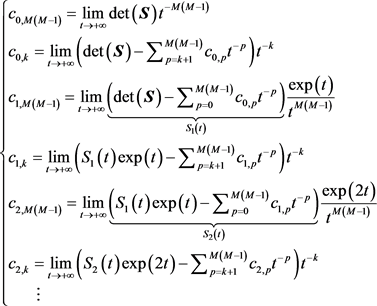(57)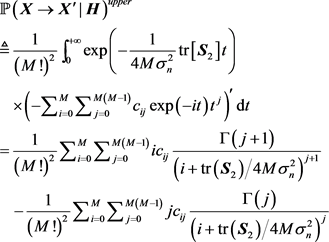(58)

5.3. 遍历化信道容量(59)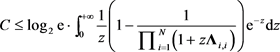(60)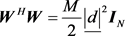(61)(62)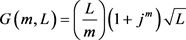(63)(64)(65)

5.4. 中断概率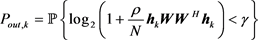(66)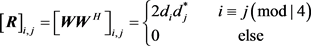(67)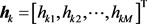满足：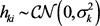。利用文献  的结果，我们有：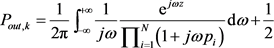(68)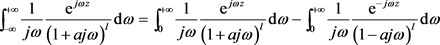(69)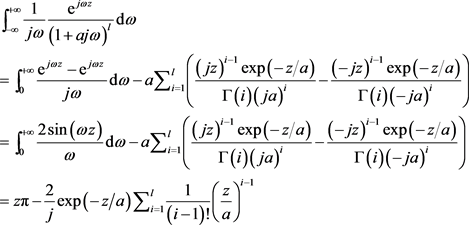(70)(71)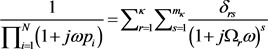(72)(73)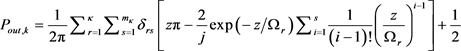(74)

6. 仿真结果(75)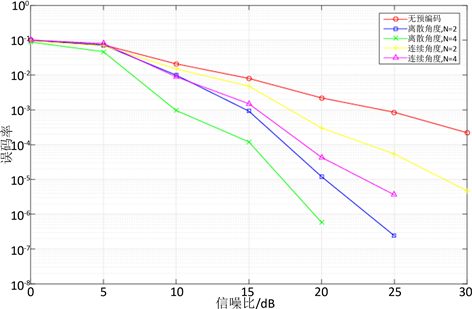Figure 2. Bit Error Rate (BER) Simulation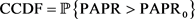(76)

7. 结论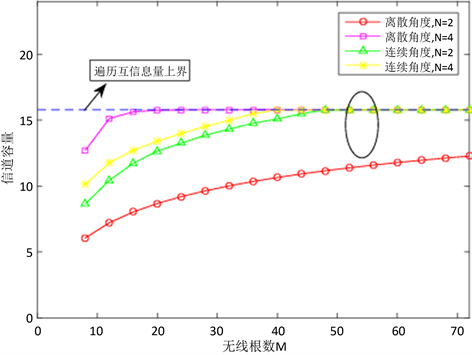Figure 3. Ergodic channel capacity versus the number of antennas for different coding schemes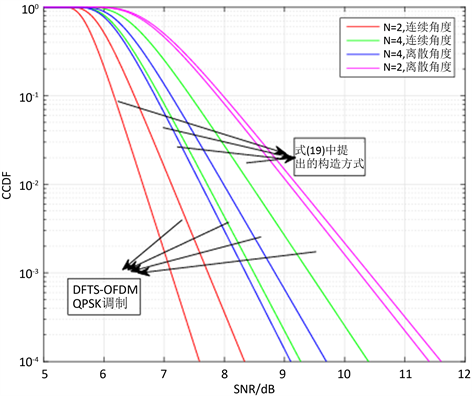Figure 4. Peak-to-average rate (PAPR) for different coding/modulation schemes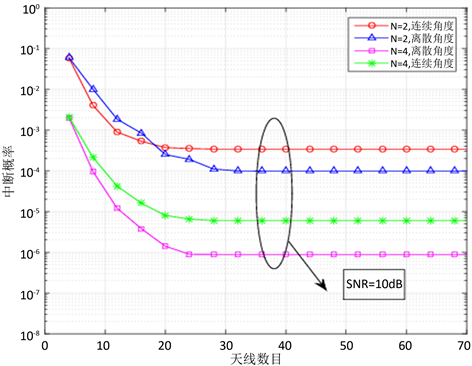Figure 5. Comparison of outage probability for different number of antennas

 戚晨皓, 黄永明, 金石. 大规模MIMO系统研究进展[J]. 数据采集与处理, 2015, 30(3): 544-551.

 Al-Askery, A.J., Tsimenidis, C.C., Boussakta, S. and Chambers, J.A. (2017) Performance Analysis of Coded Massive MIMO-OFDM Systems Using Effective Matrix Inversion. IEEE Transactions on Communications, 65, 5244-5256.
https://doi.org/10.1109/TCOMM.2017.2749370

 Suganya, M. and Gandhiraj, R. (2016) Applicability of MIMO and OFDM Technology to SATCOM. 2016 International Conference on Communication and Signal Processing (ICCSP), Melmaruvathur, 6-8 April 2016, 916-920.

 Yang, L., Zeng, Y. and Zhang, R. (2017) Channel Estimation for Millimeter Wave MIMO Communications with Lens Antenna Arrays. IEEE Transactions on Vehicular Technology, PP(99): 1-1.

 Li, T., Wang, X., Fan, P. and Riihonen, T. (2017) Position-Aided Large-Scale MIMO Channel Estimation for High-Speed Railway Communication Systems. IEEE Transactions on Vehicular Technology, 66, 8964-8978.
https://doi.org/10.1109/TVT.2017.2703595

 Yu, Z.H., Li, J., Zhang, Y.H. and Liao, G.S. (2017) Mainlobe Interference Suppression via Transmit Phase Codes Optimisation in MIMO Radar Systems. Electronics Letters, 53, 1427-1429.
https://doi.org/10.1049/el.2017.2787

 Komulainen, P., Tolli, A., M. Latva-aho and Juntti, M. (2009) Channel Sounding Pilot Overhead Reduction for TDD Multiuser MIMO Systems. 2009 IEEE Globecom Workshops, Honolulu, HI, 30 November-4 December 2009, 1-6.
https://doi.org/10.1109/GLOCOMW.2009.5360756

 Hu, A. and Pan, P. (2017) Concavity Approximation Based Power Allocation in Millimeter-Wave MIMO Systems. IEEE Access, 5, 25731-25740.
https://doi.org/10.1109/ACCESS.2017.2773491

 Alamouti, S.M. (1998) A Simple Transmit Diversity Technique for Wireless Communications. IEEE Journal on Selected Areas in Communications, 16, 1451-1458.
https://doi.org/10.1109/49.730453

 李秋萍. MIMO系统中的空时编码技术研究[D]: [硕士学位论文]. 南京: 南京邮电大学, 2010.

 Karlsson, M. and Larsson, E.G. (2014) On the Operation of Massive MIMO with and without Transmitter CSI. IEEE SPAWC, Toronto, ON, 1-5.

 Meng, X., Gao, X.Q. and Xia, X.-G. (2016) Omnidirectional Precoding Based Transmission in Massive MIMO Systems. IEEE Transactions on Communications, 64, 174-186.
https://doi.org/10.1109/TCOMM.2015.2498159

 Meng, X., Xia, X.G. and Gao, X. (2014) Constant-Envelope Omni-Directional Transmission with Diversity in Massive MIMO Systems. 2014 IEEE Global Communications Conference, Austin, TX, 3784-3789.

 Dighe, P.A., Mallik, R.K. and Jamuar, S.S. (2003) Analysis of Transmit-Receive Diversity in Rayleigh Fading. IEEE Transactions on Communications, 51, 694-703.
https://doi.org/10.1109/TCOMM.2003.810871

 Hamdi, K. (2008) Capacity of MRC on Correlated Rician Fading Channels. IEEE Transactions on Communications, 56, 708-711.
https://doi.org/10.1109/TCOMM.2008.060381

 Popovic, B.M. (2010) Efficient DFT of Zadoff-Chu Se-quences. Electronics Letters, 46, 502-503.
https://doi.org/10.1049/el.2010.3510

 Jorswieck, E.A. and Boche, H. (2007) Outage Probability in Multiple Antenna Systems. Transactions on Emerging Telecommunications Technologies, 18, 217-233.
https://doi.org/10.1002/ett.1135

Top